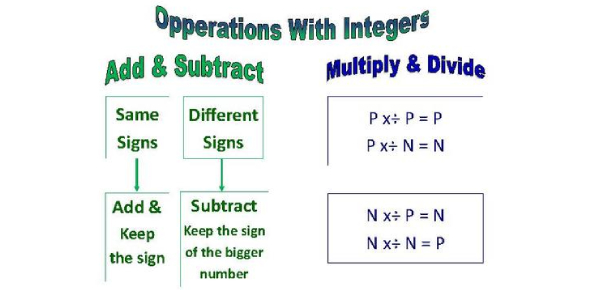# Integer Operations Quiz! Math Trivia

13 Questions | Attempts: 2433
ShareSettingsThis is an Integer Operation in Math Trivia. There are four mathematical operations, which include multiplication, addition, subtraction, and division. The simple rules followed when dealing with whole numbers change when it comes to entire negative numbers, either alone or with whole numbers in a question. This quiz will help you to be better equipped when it comes to solving these questions. Do give it a shot and get to up your skills.

• 1.
- 243 + 18=
• A.

-225

• B.

-261

• C.

225

• D.

261

• 2.
196 + (-8) =
• A.

-204

• B.

204

• C.

-188

• D.

188

• 3.
19 - (-5) =
• A.

24

• B.

-24

• C.

14

• D.

-14

• 4.
-81 - (-3) =
• A.

-84

• B.

78

• C.

-78

• D.

84

• 5.
-48 - 12=
• A.

60

• B.

-60

• C.

-36

• D.

36

• 6.
24(-8)=
• A.

192

• B.

-192

• C.

3

• D.

-3

• 7.
-164/8
• A.

-20.4

• B.

20.4

• C.

20.5

• D.

-20.5

• 8.
-8 x (-15)=
• A.

120

• B.

-120

• C.

130

• D.

-130

• 9.
52 (-13)=
• A.

4

• B.

-4

• C.

39

• D.

-38

• 10.
-18 + 5 - (-7) + 3
• A.

-17

• B.

33

• C.

-3

• D.

17

• 11.
5 - 8 + 3 + (-17)
• A.

-11

• B.

17

• C.

-17

• D.

11

• 12.
Evaluate x - 2y  + 2x - y    When x = 1 and y = 2
• A.

-9

• B.

3

• C.

-1

• D.

-3

• 13.
(-13t + 8z) + (-24t - 9z)
• A.

-37t + z

• B.

-37t - z

• C.

-14t -z

• D.

-14t + z

## Related TopicsBack to top
×

Wait!
Here's an interesting quiz for you.